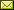## Satisfiability vs. Finite Satisfiability in Elementary Modal Logics

 Jakub Michaliszyn Jan Otop Piotr Witkowski

 We study elementary modal logics, i.e. modal logic considered over first-order definable classes of frames. The classical semantics of modal logic allows infinite structures, but often practical applications require to restrict our attention to finite structures. Many decidability and undecidability results for the elementary modal logics were proved separately for general satisfiability and for finite satisfiability [11, 12, 16, 17]. In this paper, we show that there is a reason why we must deal with both kinds of satisfiability separately – we prove that there is a universal first-order formula that defines an elementary modal logic with decidable (global) satisfiability problem, but undecidable finite satisfiability problem, and, the other way round, that there is a universal formula that defines an elementary modal logic with decidable finite satisfiability problem, but undecidable general satisfiability problem.

In Marco Faella and Aniello Murano: Proceedings Third International Symposium on Games, Automata, Logics and Formal Verification (GandALF 2012), Napoli, Italy, September 6-8, 2012, Electronic Proceedings in Theoretical Computer Science 96, pp. 141–154.
Published: 7th October 2012.

 ArXived at: http://dx.doi.org/10.4204/EPTCS.96.11 bibtex PDF
References in reconstructed bibtex, XML and HTML format (approximated).
 Comments and questions to:eptcs@eptcs.org For website issues:webmaster@eptcs.org